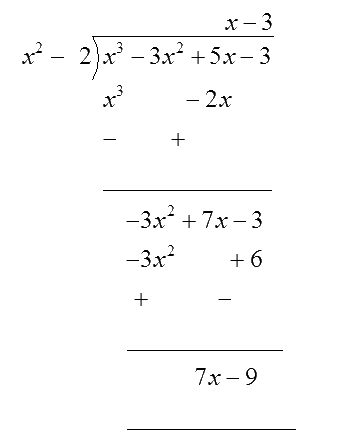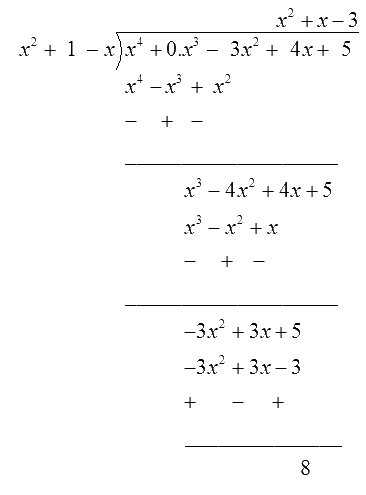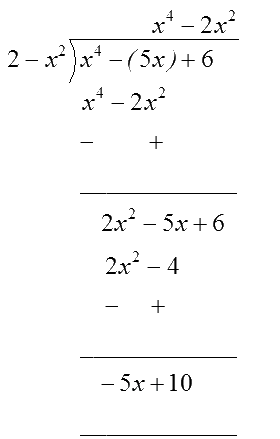# Ex.2.3 Q1 Polynomials Solution - NCERT Maths Class 10

Go back to  'Ex.2.3'

## Question

Divide the polynomial $$p(x)$$ by the polynomial $$g(x)$$ and find the quotient and remainder in each of the following:

(i)

\begin{align}\,\,\,p(x) &= {x^3} - 3{x^2} + 5x - 3, \\ \quad\;\; g(x) &= {x^2} - 2\end{align}

(ii)

\begin{align}\,\,\,p(x)& = {x^4} - 3{x^2} + 4x + 5, \\ \quad\; g(x) &= {x^2} + 1 - x\end{align}

(iii)

\begin{align}\,\,\,p(x) &= {x^4} - 5x + 6, \qquad \\ \quad g(x) &= 2 - {x^2}\end{align}

Video Solution
Polynomials
Ex 2.3 | Question 1

## Text Solution

What is unknown?

The quotient and remainder of the given polynomials.

Reasoning:

You can solve this question by following the steps given below:

First, arrange the divisor as well as dividend individually in decreasing order of their degree of terms.

In case of division, we seek to find the quotient. To find the very first term of the quotient, divide the first term of the dividend by the highest degree term in the divisor.

Now write the quotient.

Multiply the divisor by the quotient obtained. Put the product underneath the dividend.

Subtract the product obtained as happens in case of a division operation.

Write the result obtained after drawing another bar to separate it from prior operations performed.

Bring down the remaining terms of the dividend.

Again, divide the dividend by the highest degree term of the remaining divisor.

Repeat the previous three steps on the interim quotient.

Steps:

(i)

\begin{align}\,\,\,p(x) &= {x^3} - 3{x^2} + 5x - 3, \\ \quad\;\; g(x) &= {x^2} - 2\end{align}Quotient $$= x - 3,$$   Remainder $$= 7x - 9$$

(ii)

\begin{align}\,\,\,p(x)& = {x^4} - 3{x^2} + 4x + 5, \\ \quad\; g(x) &= {x^2} + 1 - x\end{align}

$={x}^{4}+0.{x}^{3}-3{{x}^{2}}+4x+5$Quotient = $${x^2} + x - 3$$   Remainder $$= 8$$

(iii)

\begin{align}\,\,\,p(x) &= {x^4} - 5x + 6, \qquad \\ \quad g(x) &= 2 - {x^2}\end{align}

\begin{align}= {x^4} + 0.{x^2}-5x + {\text{ }}6\end{align}Quotient $$= - {x^2} - 2,$$   Remainder $$= - 5x + 10$$

Learn from the best math teachers and top your exams

• Live one on one classroom and doubt clearing
• Practice worksheets in and after class for conceptual clarity
• Personalized curriculum to keep up with school